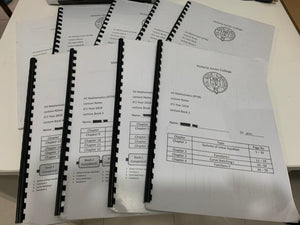# VJC H2 Math Lecture notes 2018-2019 ( JC1 & JC2 ) ( New addition )

Regular price
\$35.00
Sale price
\$35.00
Unit price
per

JC1 and JC2 Lecture notes (annotated with nice handwriting) -- HIGH QUALITY

Spent literally hours to scan the materials.

Booklet 1 to 9 (each booklet \$5) (Purchase all \$35)

Book 1:

• Systems of Linear Equation
• Functions l
• Curve Sketching l
• Functions ll

Book 2:

• Inequalities
• Arithmetic Progression
• Geometric Progression
• Summation & Method  of Differences

Book 3:

• Techniques of Differentiation
• Applications of Differentiation
• Transformation of Graphs
• Conics

Book 4:

• Series Expansion
• Curve Sketching 2
• Deduced Graphs
• Complex Numbers

Book 5:

• Techniques of Integration
• Area
• Volume of Revolution

Book 6:

• Differential Equations
• Permutations and Combinations
• Probability

Book 7:

• Vectors 1
• Vectors 2
• Vectors 3
• Vectors 4
• Vectors 5
• Vectors 6

Book 8:

• Discrete Random Variables
• Binomial Distribution
• Normal Distribution
• Sampling Distribution

Book 9:

• Hypothesis Testing
• Correlation and Linear Regression